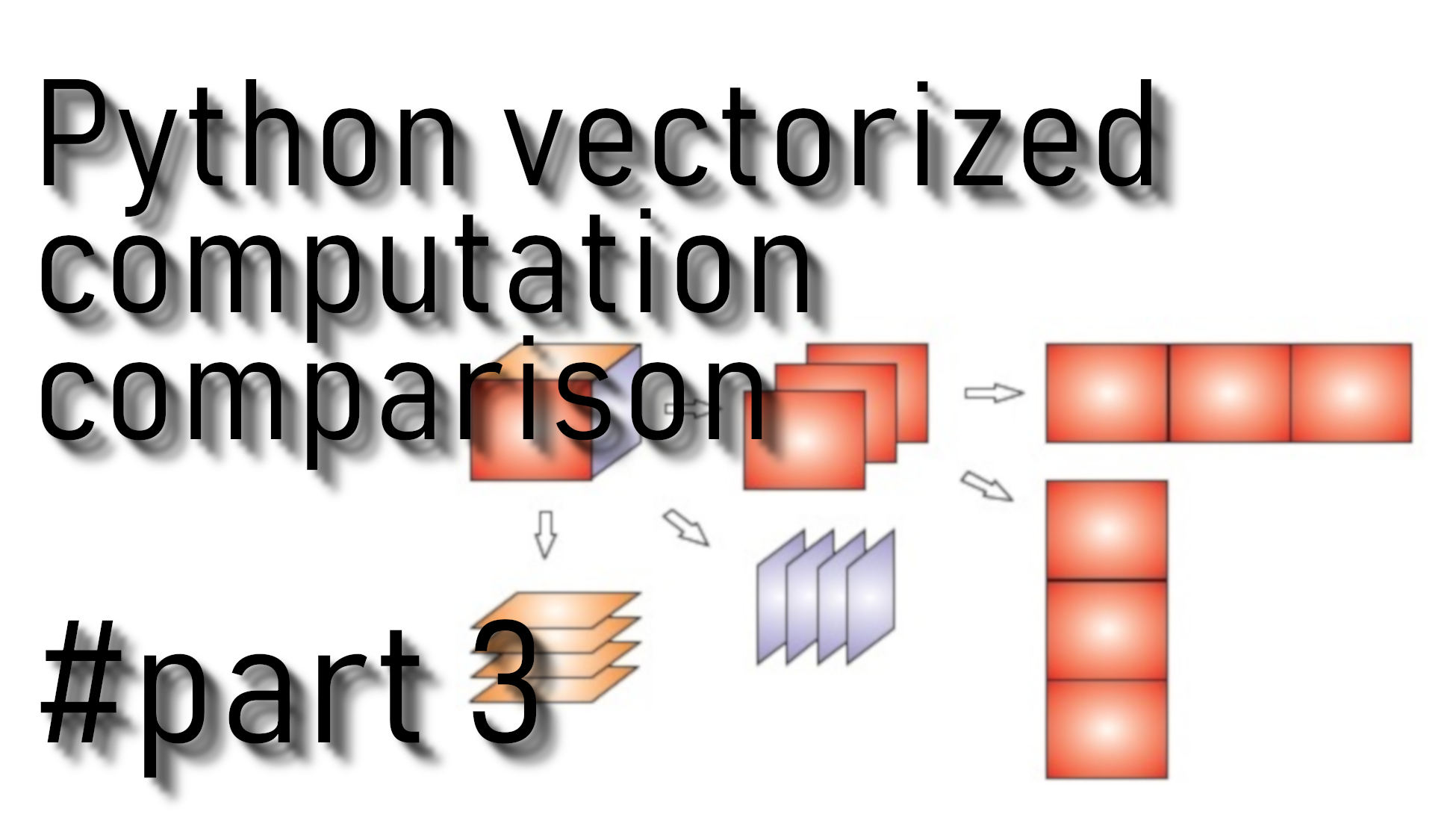# Vectorized and non vectorized mathematical computations

## When we are writing machine learning functions, we must be sure that our code is computationally efficient so we always use vectorization

In deep learning, we usually deal with huge datasets. When we are writing machine learning functions, we must be sure that our code is computationally efficient, so we always use vectorization. A non-computationally optimal function can become a huge bottleneck in our algorithm and can result in a model that takes ages to run.

To compare vectorized and not vectorized computation time, we create random numbers between 11 and 99. We create two lists:

``````x1 = np.random.randint(low=11, high=99, size=10000)
x2 = np.random.randint(low=11, high=99, size=10000)``````

## Let's compare DOT implementation with for loops and NumPy library:

``````# CLASSIC DOT VECTORS IMPLEMENTATION
tic = time.time()
dot = 0
for i in range(len(x1)):
dot+= x1[i]*x2[i]
toc = time.time()
print("Computation time = ",1000*(toc - tic))

# VECTORIZED DOT IMPLEMENTATION
tic = time.time()
dot = np.dot(x1,x2)
toc = time.time()
print("Computation time = ",1000*(toc - tic))``````

We tried the above code example with examples size=10000. Results were:
Computation time  not vectorized: 234.51972007751465
Computation time vectorized: 0.9977817535400391
From our results, we can tell that `np.dot` is faster more than 200 times.

## Let's compare OUTER implementation with for loops and NumPy library:

``````# CLASSIC OUTER IMPLEMENTATION
tic = time.time()
outer = np.zeros((len(x1),len(x2))) # we create a len(x1)*len(x2) matrix with only zeros
for i in range(len(x1)):
for j in range(len(x2)):
outer[i,j] = x1[i]*x2[j]
toc = time.time()
print("Computation time = ",1000*(toc - tic))

# VECTORIZED OUTER IMPLEMENTATION
tic = time.time()
outer = np.outer(x1,x2)
toc = time.time()
print("Computation time = ",1000*(toc - tic))``````

We tried the above code example with examples size=1000. Results were:
Computation time = 747.028112411499
Computation time = 3.990650177001953
From our results, we can tell that `np.outer` is faster, close to 200 times.

## Let's compare ELEMENTWISE multiplication implementation with for loops and NumPy library:

``````# CLASSIC ELEMENTWISE IMPLEMENTATION
tic = time.time()
mul = np.zeros(len(x1))
for i in range(len(x1)):
mul[i] = x1[i]*x2[i]
toc = time.time()
print("Computation time = ",1000*(toc - tic))

# VECTORIZED ELEMENTWISE IMPLEMENTATION
tic = time.time()
mul = np.multiply(x1,x2)
toc = time.time()
print("Computation time = ",1000*(toc - tic))``````

We tried the above code example with examples size=500000. Results were:
Computation time = 334.1071605682373
Computation time = 1.9948482513427734
From our results, we can tell that `np.multiply` is faster, around 150 times.

## Let's compare general DOT implementation with for loops and NumPy library:

``````# CLASSIC GENERAL DOT IMPLEMENTATION
W = np.random.rand(3,len(x1)) # Random 3*len(x1) numpy array
tic = time.time()
gdot = np.zeros(W.shape)
for i in range(W.shape):
for j in range(len(x1)):
gdot[i] += W[i,j]*x1[j]
toc = time.time()
print("Computation time = ",1000*(toc - tic))

# VECTORIZED GENERAL DOT IMPLEMENTATION
tic = time.process_time()
dot = np.dot(W,x1)
toc = time.process_time()
print("Computation time = ",1000*(toc - tic))``````

We tried the above code example with examples size=500000. Results were:
Computation time = 1468.2056903839111
Computation time = 3.125
From our results, we can tell that the general `np.dot` is faster, around 500 times.

As you may have noticed, the vectorized implementation is much cleaner and more efficient. For bigger vectors and matrices, the differences in running time become even bigger.

## Conclusion:

Keep in mind that vectorization is very important in deep learning. It provides computational efficiency and clarity.
We know what is sigmoid, sigmoid derivatives, array reshaping, rows normalization, broadcasting, softmax, and vectorization. So in the next tutorial, we will start building a gradient descent function where everything will be more exciting and interesting! I hope that we have a little warm-up that will help you in the future up to this point.